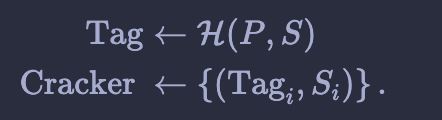﻿ 密码学系列之:memory-hard函数
﻿• 价格透明
• 信息保密
• 进度掌控
• 售后无忧# 密码学系列之:memory-hard函数

### 文章目录

• 简介
• 为什么需要MHF
• Memory hard的评估方法
• MHF的种类
• MHF的密码学意义
• memory-hard在MHF中的应用

# 简介

Memory hard function简称为MHF，在密码学中，内存困难函数(MHF)是一个需要花费大量内存来完成的函数。MHF主要被用在工作量证明中。因为需要花费大量的内存，所以MHF也会被用在密码Hash中，可以防止恶意破解。

# MHF的种类

dMHFs的例子是scrypt和Argon2d。iMHFs的例子是Argon2i和catena。

# MHF的密码学意义E max ⁡ = max ⁡ α 1 α D ( α ) \mathcal{E}_{\max }=\max _{\alpha} \frac{1}{\alpha D(\alpha)}

## memory-hard在MHF中的应用

B [ j ] = G ( B [ ϕ 1 ( j ) ] , B [ ϕ 2 ( j ) ] , ⋯   , B [ ϕ k ( j ) ] ) B[j]=G\left(B\left[\phi_{1}(j)\right], B\left[\phi_{2}(j)\right], \cdots, B\left[\phi_{k}(j)\right]\right)

E ( α ) = 1 α + C ( α ) β \mathcal{E}(\alpha)=\frac{1}{\alpha+C(\alpha) \beta}

E ( α ) = 1 ( α + C ( α ) β ) D ( α ) \mathcal{E}(\alpha)=\frac{1}{(\alpha+C(\alpha) \beta) D(\alpha)}### 低价透明### 金牌服务### 信息保密